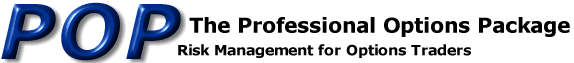Online Options Help
Risk Projections

 The position projections show your theoretical risk over a range of prices in the underlying. There may also be additional projections that show the position's risk at some point in the future or at a different volatility. If you want the additional projections, but do not see them, be sure to enter values in the Days Increment and Volatility Increment boxes. Theoretical Value: What is the option worth according to the theoretical pricing model. The various models require five standard inputs in order to generate theoretical prices. The inputs are: the price of the underlying instrument, the strike price, the number of days remaining until expiration, the volatility of the underlying contract, and the interest rate. There are various methods used to determine each of the above. It is beyond the scope of this paper to explain them here. However, most floor traders use current market values. These are available at PMpublishing's Daily Options Summary. Theta: How much the position loses if the number of days is decreased by 1. A negative number means that you are short the option and expect to make money overnight. Vega: How much the position makes if the volatility increases by 1%. Gamma: How much the delta changes with a one point move in the underlying. Delta: How much does the option price changes with a one point move in the underlying. P/L: What is the theoretical profit (or loss) of the position as the underlying moves? This value is displayed in addition to the delta since the ganmma (2nd derivative) effect causes the delta to be accurate over a narrow price range only. Online Pricing Input Pagee-mail: info@pmpublishing.com